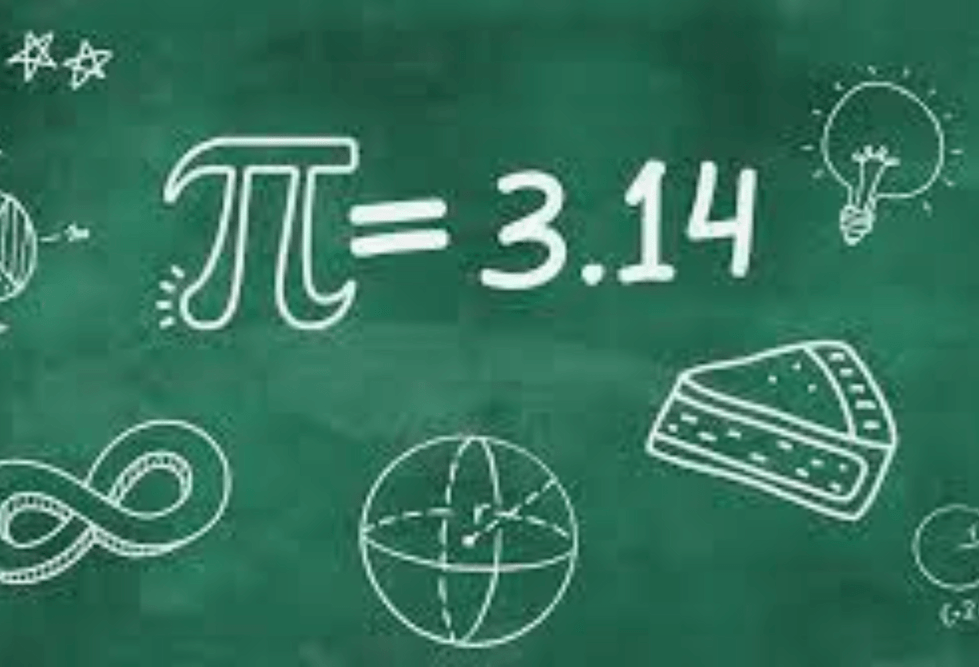### Pi (π) Day

14 Mar 2024

Pi Day is an annual celebration of the mathematical constant pi (π). It is observed on March 14th (3/14 in the month/day format) since 3, 1, and 4 are the first three significant digits of pi. Pi is the ratio of the circumference of a circle to its diameter, and it's an irrational number (meaning it cannot be expressed as a simple fraction). It has been studied and used for thousands of years and has many applications in mathematics, science, and engineering.

Pi Day is a fun and educational celebration for students. Teachers and students engage in various activities, from Pi Day Scavenger Hunt to a pi-themed trivia game, to enjoy learning about and appreciating the mathematical constant pi.